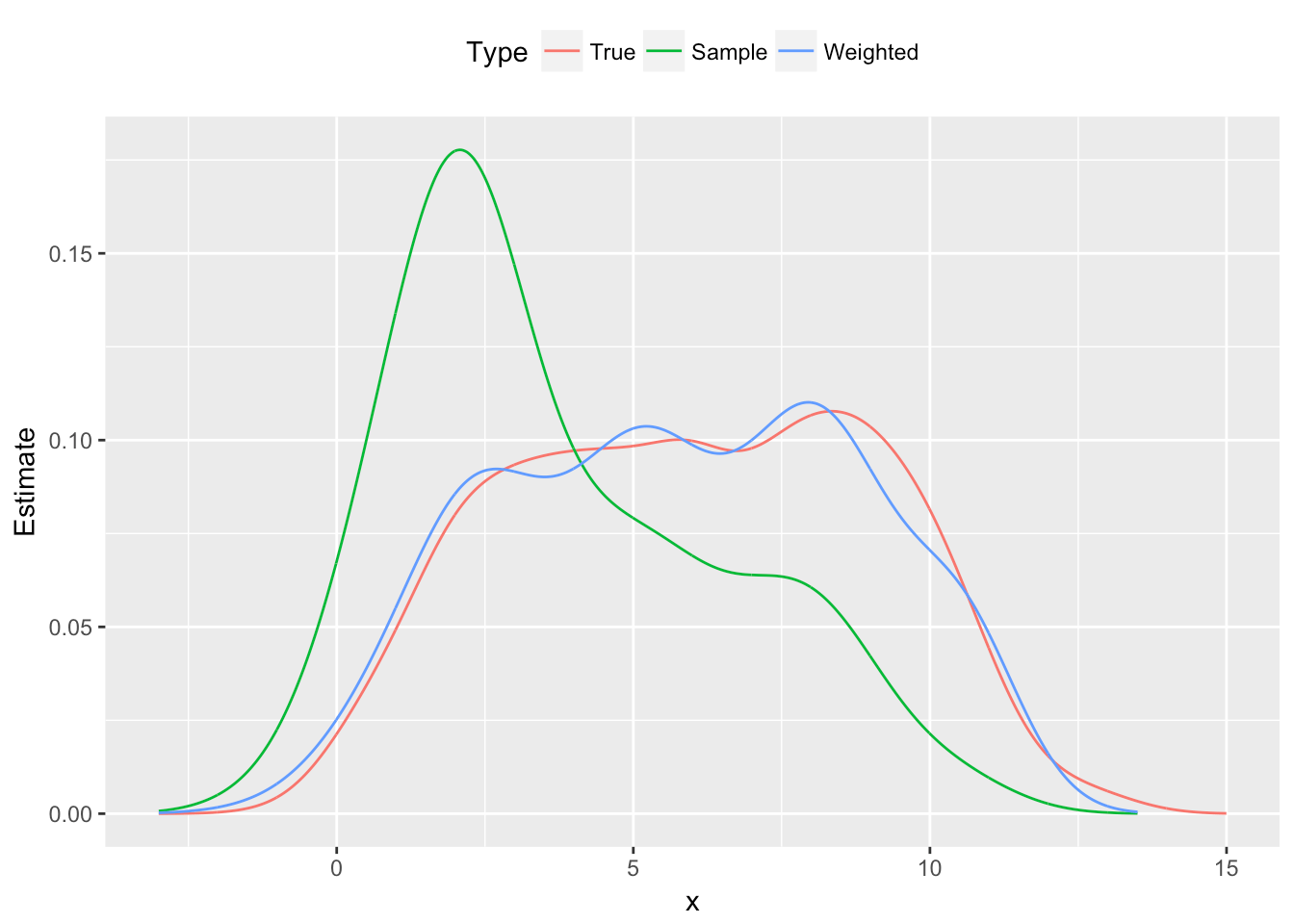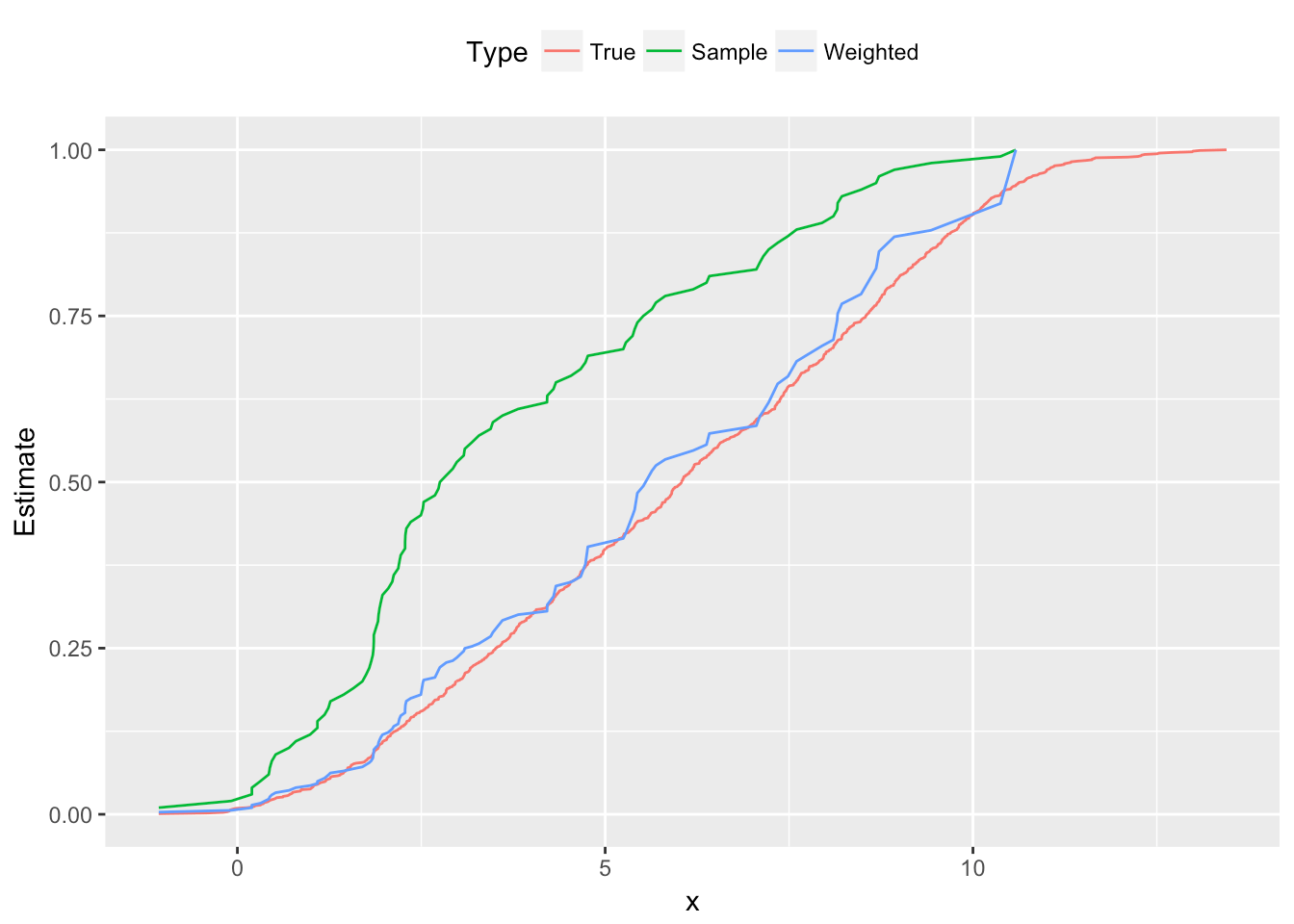Want to share your content on R-bloggers? click here if you have a blog, or here if you don't.

In our first blog post, we introduced CVXR, an R package for disciplined convex optimization, and showed how to model and solve a non-negative least squares problem using its interface. This time, we will tackle a non-parametric estimation example, which features new atoms as well as more complex constraints.

## Direct Standardization

Consider a set of observations $$(x_i,y_i)$$ drawn non-uniformly from an unknown distribution. We know the expected value of the columns of $$X$$, denoted by $$b \in {\mathbf R}^n$$, and want to estimate the true distribution of $$y$$. This situation may arise, for instance, if we wish to analyze the health of a population based on a sample skewed toward young males, knowing the average population-level sex, age, etc.

A naive approach would be to simply take the empirical distribution that places equal probability $$1/m$$ on each $$y_i$$. However, this is not a good estimation strategy when our sample is unbalanced. Instead, we will use the method of direct standardization (Fleiss, Levin, and Paik 2003, 19.5): we solve for weights $$w \in {\mathbf R}^m$$ of a weighted empirical distribution, $$y = y_i$$ with probability $$w_i$$, which rectifies the skewness of the sample. This can be posed as the convex optimization problem

$\begin{array}{ll} \underset{w}{\mbox{maximize}} & \sum_{i=1}^m -w_i\log w_i \\ \mbox{subject to} & w \geq 0, \quad \sum_{i=1}^m w_i = 1,\quad X^Tw = b. \end{array}$

Our objective is the total entropy, which is concave on $${\mathbf R}_+^m$$. The constraints ensure $$w$$ is a probability vector that induces our known expectations over the columns of $$X$$, i.e., $$\sum_{i=1}^m w_iX_{ij} = b_j$$ for $$j = 1,\ldots,n$$.

## An Example with Simulated Data

As an example, we generate $$m = 1000$$ data points $$x_{i,1} \sim \mbox{Bernoulli}(0.5)$$, $$x_{i,2} \sim \mbox{Uniform}(10,60)$$, and $$y_i \sim N(5x_{i,1} + 0.1x_{i,2},1)$$. We calculate $$b_j$$ to be the mean over $$x_{.,j}$$ for $$j = 1,2$$. Then we construct a skewed sample of $$m = 100$$ points that over-represent small values of $$y_i$$, thus biasing its distribution downwards.

Using CVXR, we construct the direct standardization problem. We first define the variable $$w$$.

w <- Variable(m)

Then, we form the objective function by combining CVXR’s library of operators and atoms.

objective <- Maximize(sum(entr(w)))

Here, entr is the element-wise entropy atom; the S4 object entr(w) represents an $$m$$-dimensional vector with entries $$-w_i\log(w_i)$$ for $$i=1,\ldots,m$$. The sum operator acts exactly as expected, forming an expression that is the sum of the entries in this vector. (For a full list of atoms, see the function reference page).

Our next step is to generate the list of constraints. Note that, by default, the relational operators apply over all entries in a vector or matrix.

constraints <- list(w >= 0, sum(w) == 1, t(X) %*% w == b)

Finally, we are ready to formulate and solve the problem.

prob <- Problem(objective, constraints)
result <- solve(prob)
weights <- result$getValue(w) Using our optimal weights, we can then re-weight our skewed sample and compare it to the population distribution. Below, we plot the density functions using linear approximations for the range of $$y$$. ## Approximate density functions dens1 <- density(ypop) dens2 <- density(y) dens3 <- density(y, weights = weights) yrange <- seq(-3, 15, 0.01) d <- data.frame(x = yrange, True = approx(x = dens1$x, y = dens1$y, xout = yrange)$y,
Sample = approx(x = dens2$x, y = dens2$y, xout = yrange)$y, Weighted = approx(x = dens3$x, y = dens3$y, xout = yrange)$y)

## Plot probability distribution functions
plot.data <- gather(data = d, key = "Type", value = "Estimate", True, Sample, Weighted,
factor_key = TRUE)
ggplot(plot.data) +
geom_line(mapping = aes(x = x, y = Estimate, color = Type)) +
theme(legend.position = "top")
## Warning: Removed 300 rows containing missing values (geom_path).Figure 1: Probability distribution functions: population, skewed sample and reweighted sample

## Return the cumulative distribution function
get_cdf <- function(data, probs, color = 'k') {
if(missing(probs))
probs <- rep(1.0/length(data), length(data))
distro <- cbind(data, probs)
dsort <- distro[order(distro[,1]),]
ecdf <- base::cumsum(dsort[,2])
cbind(dsort[,1], ecdf)
}

## Plot cumulative distribution functions
d1 <- data.frame("True", get_cdf(ypop))
d2 <- data.frame("Sample", get_cdf(y))
d3 <- data.frame("Weighted", get_cdf(y, weights))

names(d1) <- names(d2) <- names(d3) <- c("Type", "x", "Estimate")
plot.data <- rbind(d1, d2, d3)

ggplot(plot.data) +
geom_line(mapping = aes(x = x, y = Estimate, color = Type)) +
theme(legend.position = "top")Figure 2: Cumulative distribution functions: population, skewed sample and reweighted sample

As is clear from the plots, the sample probability distribution peaks around $$y = 2.0$$, and its cumulative distribution is shifted left from the population’s curve, a result of the downward bias in our sampled $$y_i$$. However, with the direct standardization weights, the new empirical distribution cleaves much closer to the true distribution shown in red.

We hope you’ve enjoyed this demonstration of CVXR. For more examples, check out our official site and recent presentation “Disciplined Convex Optimization with CVXR” at useR! 2018.